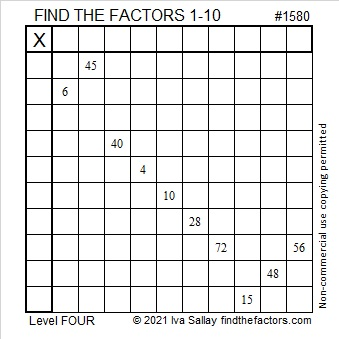# 1580 Use Logic to Solve This Puzzle

Contents

### Today’s Puzzle:

Where should you write the numbers from 1 to 10 in both the first column and the top row to make this puzzle function like a multiplication table? Study it until you find a logical place to start and continue to use logic until you’ve completed the puzzle.### Factors of 1580:

• 1580 is a composite number.
• Prime factorization: 1580 = 2 × 2 × 5 × 79, which can be written 1580 = 2² × 5 × 79.
• 1580 has at least one exponent greater than 1 in its prime factorization so √1580 can be simplified. Taking the factor pair from the factor pair table below with the largest square number factor, we get √1580 = (√4)(√395) = 2√395.
• The exponents in the prime factorization are 2, 1, and 1. Adding one to each exponent and multiplying we get (2 + 1)(1 + 1)(1 + 1) = 3 × 2 × 2 = 12. Therefore 1580 has exactly 12 factors.
• The factors of 1580 are outlined with their factor pair partners in the graphic below.### More about the Number 1580:

1580 is the difference of two squares in two different ways:
396² – 394² = 1580, and
84² – 74² = 1580.

This site uses Akismet to reduce spam. Learn how your comment data is processed.